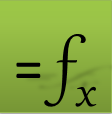# Solving Nonlinear Equations Systems in Excel

Find best answer to a system of algebraic equations

NLSOLVE is a powerful spreadsheet solver function based on the Levenberg-Marquardt algorithm which is suitable for:

1. Finding roots of nonlinear equations.

2. Computing exact or best (least-square-error) solution to a system of coupled equations including systems with mixed equations and inequalities constraints.

3. Solving least squares best curve fitting problems.

4. Solving parametrized optimization problems in conjunction with other calculus functions.

## Description

This video demonstrates how to use the function NLSOLVE to solve nonlinear equation systems in both Excel and Google Sheets. Multiple examples are illustrated including a system with inequality equation and a parameterized integral problem. NLSOLVE is based on Levenberg, Marquardt algorithm.

## Description

This video demonstrates how to use the function NLSOLVE to find least-squares best fitting curve for a set of (x,y) data points in both Excel and Google Sheets. NLSOLVE is based on Levenberg, Marquardt algorithm.
Question or Comment? Email us:
support @ excel-works.comExceLab: Transforming Excel into a Calculus Power House

ExceLab functions and methods are protected by USA Patents 10628634, 10114812, 9892108 and 9286286.